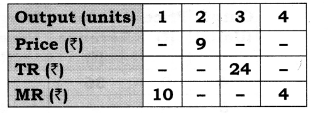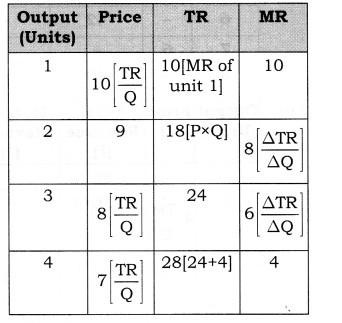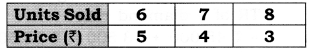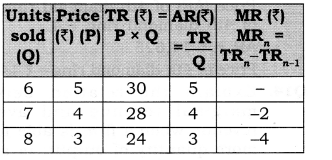## NCERT Solutions for Class 12 Micro Economics Chapter 8 Revenue

### NCERT TEXTBOOK QUESTIONS SOLVED

Question 1. How are the total revenue of a firm, market price, and the quantity sold by the firm related to each other?[1 Mark]
Answer:  Total Revenue = Market Price x Quantity sold

Question 2. Compute the total revenue, marginal revenue and average revenue schedules in the following table. Market price of each unit of good is Rs. 10.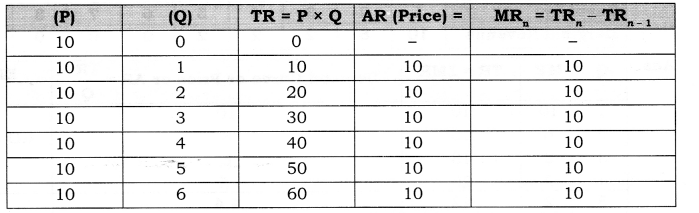Question 3. What would be the shape of the demand curve so that the total revenue curve is
(a) A positively sloped straight line passing through the origin?
(b) A horizontal line?[3-4 Marks](b) When TR is a horizontal line, demand curve is a rectangular hyperbola. It is shown: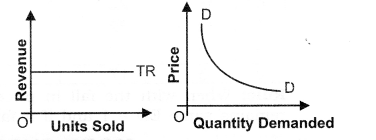The reason is that,the price at each level of output declines.

Question 4. Comment on the shape of the MR curve in case the TR curve is a (i) positively sloped straight line, (ii) horizontal straight line. [3-4 Marks]
Answer:  (i) When TR curve is positively sloped straight line, MR is a horizontal line. MR coincides with the demand curve. Price or AR is constant at each level of output.When AR is constant, MR is also constant.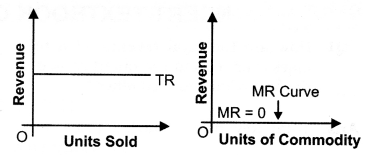(ii) When TR is a horizontal straight line, MR is zero. It is because horizontal TR means when price falls, quantity demanded rises in the same proportion. Thus, MR is zero. MR curve coincides with the x-axis

Question 5. From the schedule provided below calculate the total revenue, demand curve and the price elasticity of demand: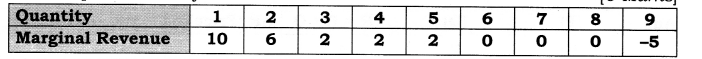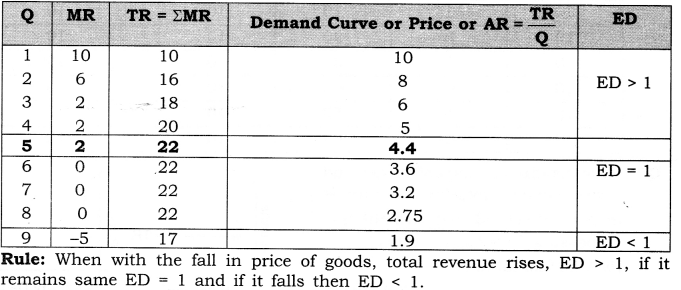### MORE QUESTIONS SOLVED

I. Very Short Answer Type Questions (1 Mark)
Question 1. Give the meaning of revenue. Or [CBSE 2007]
Define revenue. [CBSE 2008]
Answer: Revenue of a firm refers to receipts from the sale of output in a given period.

Question 2. Define total revenue.[CBSE, All India 2004]
Answer: The amount received from the sale of given amount of output is known as Total Revenue.
For example, if a firm sells 100 chairs at a price of ? 200 per chair, The total revenue will be 100 Chairs x Rs. 200 = Rs 20,000.

Question 3. Define average revenue.[CBSE, Foreign 2004]
Answer: The per unit amount received from the sale of given amount of output.

Question 4. Define marginal revenue.
Answer: The amount received from the sale of additional unit of output is known as Marginal Revenue.

Question 5. How is MR derived from TR?
Answer: $$M{ R }_{ n }=T{ R }_{ n }-T{ R }_{ n-1 }$$

Question 6. What change in TR will result in a decrease in MR?
Answer: When TR increases at a diminishing rate.

Question 7. When TR falls, what happens to MR?

Question 8. How does TR change with the output when MR is zero?
Answer:  Then, TR is maximum and constant.

Question 9. What is the behaviour of average revenue in a market in which a firm can sell more only by lowering the price? [CBSE 2012]

Question 10. What is the behaviour of Marginal Revenue in a market in which a firm can sell any quantity of the output it produces at a given price? [AI 2012]

II. Multiple Choice Questions (1 Mark)
Question 1. Assume that when price is Rs.20, the quantity demanded is 9 units, and when price is Rs.19 the quantity demanded is 10 units. Based on this information what is the marginal revenue resulting from an increase in output from 9 units to 10 units.
(a) Rs.20 (b)Rs.19 (c) Rs.10 (d) Rs. 1

Question 2. Assume that when price is Rs.20, the quantity demanded is 15 units and when price is Rs.18, the quantity demanded is 16 units. Based on this information what is the marginal revenue resulting from an increase in output from 15 units to 16 units?
(a) Rs. 18 (b) Rs.16 (c) Rs.12 (d) Rs. 28

Question 3. Marginal Revenue is equal to:
(a) The change in price divided by the change in output.
(b) The change in quantity divided by the change in price.
(c) The change in P * Q due to a one unit change in output.
(d) Price, but only if the firm is a price searcher.

Question 4. Total revenue =
(a) Price x quantity (b) Price x income
(c) Income x quantity
(d) None of these.

Question 5. Average revenue is the revenue earned
(a) per unit of input
(b) per unit of output
(c) different units of input
(d) different units of output

Question 6. AR can be symbolically written as:
(a) MR/Q (b) Price x quantity
(c) TR/Q (d) None of these.

Question 7. AR is also known as:
(a) Price (b) Income
(c) Revenue (d) None of these.

Question 8. Marginal revenue can be defined as the change in total revenue resulting from—
(a) purchase of an additional unit of a commodity
(b) sale of an additional unit of a commodity
(c) sale of subsequent units of a product
(d) None of these.

Question 9. When price remains constant at all level of output, total revenue—
(a) increases at increasing rate
(b) increases at diminishing rate
(c) increases at constant rate
(d) None of these.

Question 10. How does TR change with output when MR is negative?
(a) TR falls with the increase in output
(b) TR rise with the increase in output
(c) TR falls with the decrease in output
(d) None of these.

Question 11. Average revenue curve is also known as:
(a) Profit Curve
(b) Demand Curve
(c) Average Cost Curve
(d) Indifference Curve

III. Short Answer Type Questions (3-4 Marks)
Question 1. Calculate Average Revenue (AR) and Marginal Revenue (MR).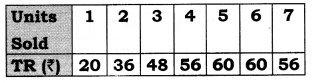Question 2. Calculate TR and AR from the following data.Question 3. Calculate TR and MR from the following data.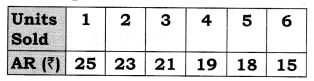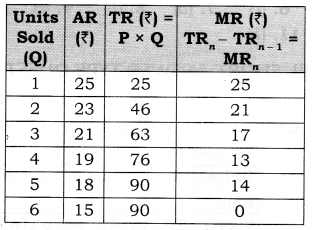Question 4. Complete the following table. [CBSE Delhi 2005 (Set-1)]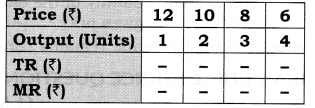Question 5. Complete the following table. [CBSE Delhi 2005 (Set-II)]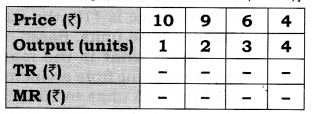Question 6. Complete the following table. [CBSE Delhi 2005 (Set-Ill)]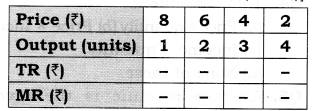Question 7. Complete the following table.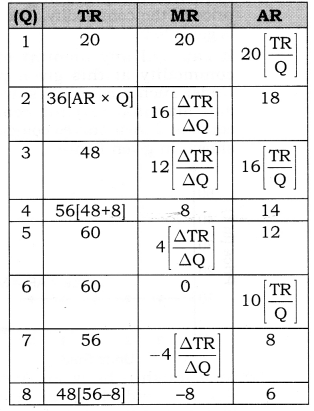Question 8. Complete the following table.Question 9. Complete the following table.